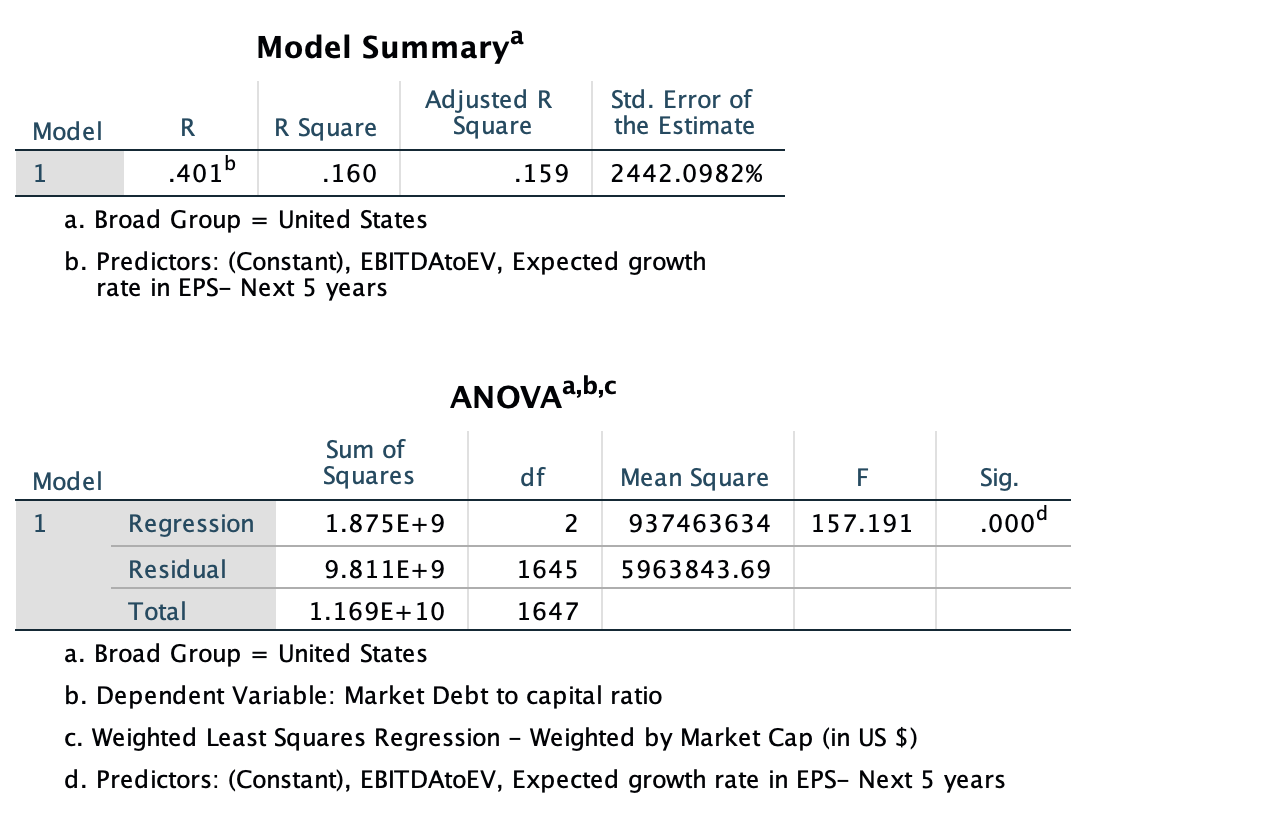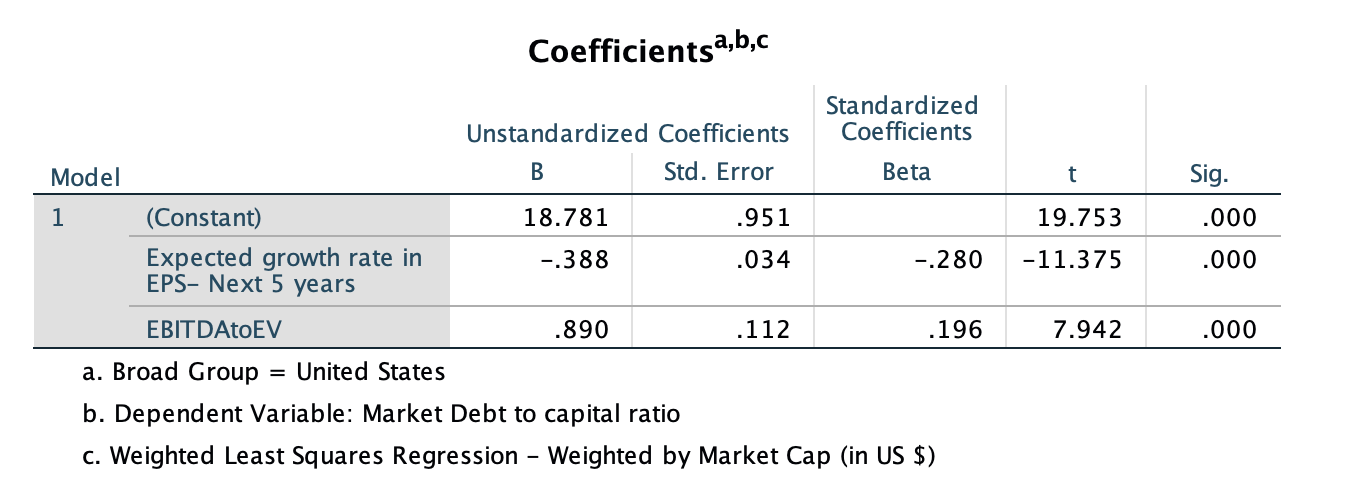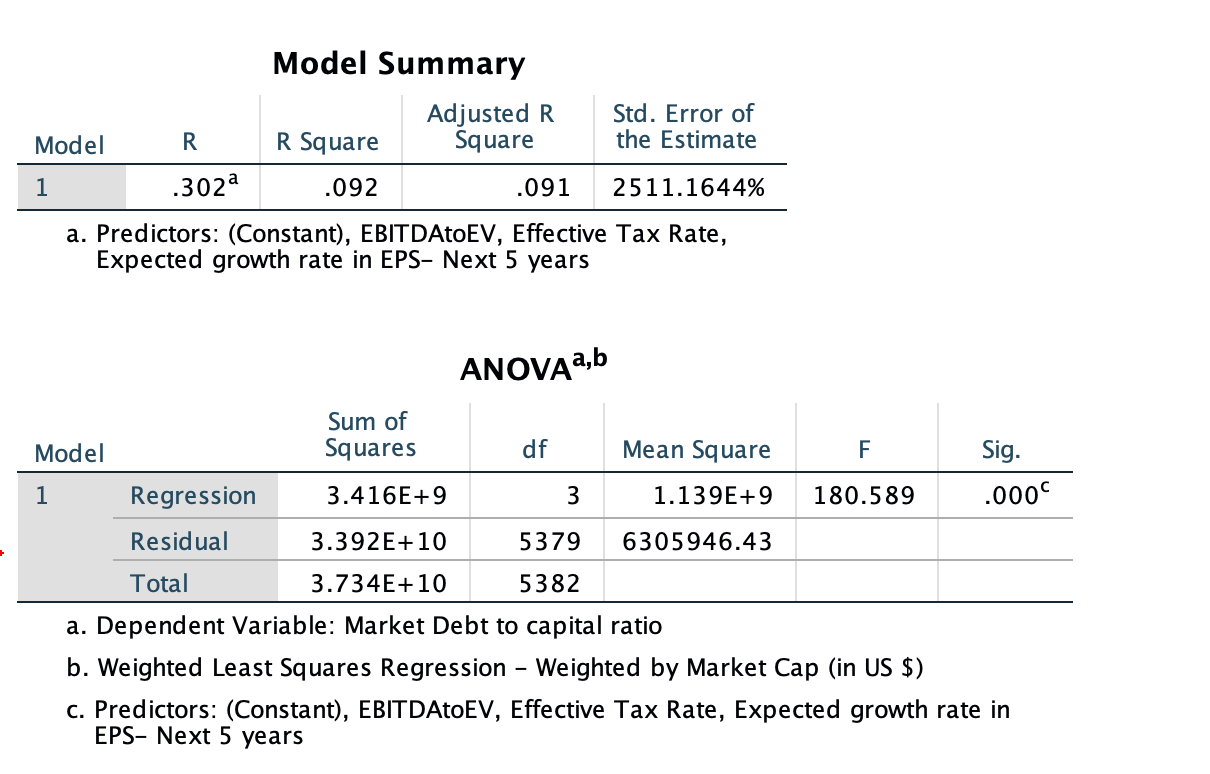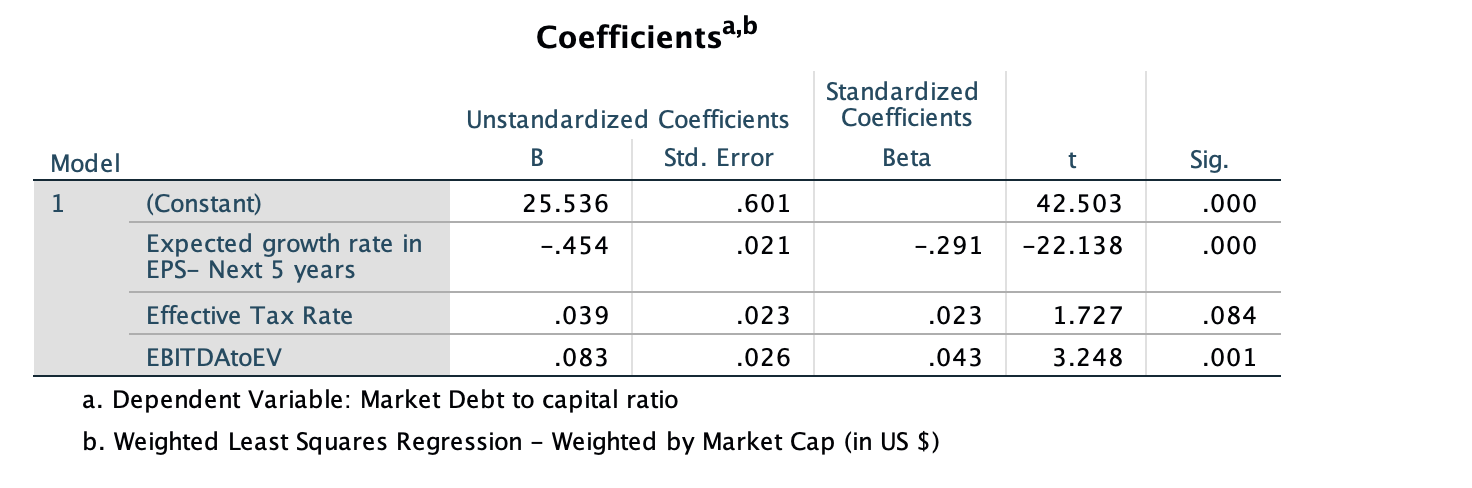Debt Ratio Regression: January 2021

Variables used in the regression

1. Debt Ratio = Debt/ (Market Value of Equity + Debt): If you can get market value of debt, use it. Else, use book value of debt.
2. Payout Ratio= Dividends/ Net Income, if Net Income is positve, not available if net income is negative.
3. Expected growth rate in EPS- next 5 years= You can use expected or even historical earnings growth, if you don't have an EPS growth forecast
4. Effective Tax Rate = Effective tax rate in most recent year

US RegressionUS RegressionGlobal RegressionGlobal Regression• How do I use this regression?

Assume that you want to estimate the market debt ratio for a firm with the following characteristics, using the Global regression

EBITDA/EV = 15%

Effective Tax Rate= 20%

Expected growth rate in EPS = 15%

• Predicted Value

Expected Debt Ratio =  25.54 - 0.454 (15) + 0.039  (.0) +  0.083 (.5)= 20.76 or 20.76%

If your predicted value is less than zero, your predicted debt ratio is zero.